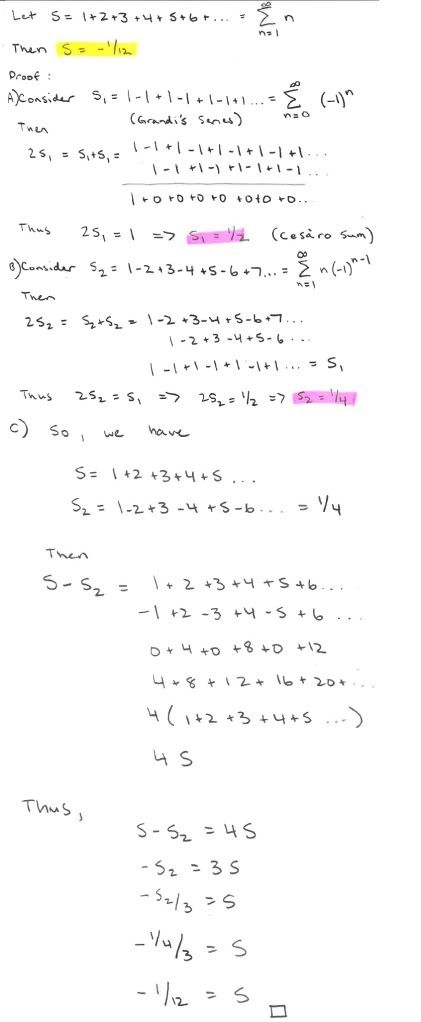# Thread: 1 + 2 + 3 + 4 + 5 + 6 + ... = -1/12

1. ## 1 + 2 + 3 + 4 + 5 + 6 + ... = -1/12

Posted this over at another forum. Thought you guys would appreciate it.

Saw a video today which reminded me of this little gem:The issues that underlie the problems with this proof are complex, shall we say. I don't want to string you guys along, but since some of you are rather analytic, continuation of this sort of cryptic verbiage will likely be welcomed.Reply With Quote

2. My mind was boggled too when I first saw this. But replace S with "infinity" and now you see the problem.
It's true that
inf -inf = 4*inf= inf = 5 *inf +10*inf = 50*inf +4*inf.

With this in mind, you can make S = any number that you want it to.Reply With Quote

3.Originally Posted by P-OMy mind was boggled too when I first saw this. But replace S with "infinity" and now you see the problem.
It's true that
inf -inf = 4*inf= inf = 5 *inf +10*inf = 50*inf +4*inf.

With this in mind, you can make S = any number that you want it to.
It isn't just S in that equation though, it's S and S2. You're presupposing that S is infinity, as well, which doesn't disprove anything; it's simply circular (or maybe not even that) reasoning. Not claiming the proof in the post above is valid, just that your reasoning for why it is invalid isn't appropriate.Reply With Quote

4. When you use the algebra you use, you're presupposing that S is NOT infinite. That's the error.Reply With Quote

5.Originally Posted by P-OWhen you use the algebra you use, you're presupposing that S is NOT infinite. That's the error.
That simply isn't true. You. Are. Mistaken. Period. I mean no offense, but I'm holding fast here. Consider refreshing your memory on valid laws for infinite limits. There are clearly defined ways of dealing with infinity in algebra, and equalities still hold true under certain operations.Reply With Quote

6. My first post shows why using the algebra you did, in the case of infinite quantities, is going to give meaningless results. If that's true, the validity of the proof rests on the fact that S is not infinite. Some moves are valid in the case of infinities, but the ones I highlighted aren't for reasons that I showed.

Either the example I gave in the first post is wrong for some reason (please show me), or you have to show that S is not infinite before using that algebra.Reply With Quote

7. It is equal to -1/12(R) not -1/12!

https://en.wikipedia.org/wiki/Ramanu...vergent_series

It is "not a sum in the traditional sense".Reply With Quote

8.Originally Posted by JohnClayIt is equal to -1/12(R) not -1/12!

https://en.wikipedia.org/wiki/Ramanu...vergent_series

It is "not a sum in the traditional sense".Technically the (R) there is simply used to denote the method of summation. That is, indeed, one way to find the sum of a divergent series. There are others, which I allude to in the OP.Reply With Quote

9. Proof: S= 1/4

S3 = S - 1
S3 = 0+1+2+3+4...

so,
S = S-1

S - S2 = 4S
S-1-S2 = 4S
1- 1/4 = 3S = 3/4
S = 1/4Reply With Quote

10.Originally Posted by P-OProof: S= 1/4

S3 = S - 1
S3 = 0+1+2+3+4...

so,
S = S-1

S - S2 = 4S
S-1-S2 = 4S
1- 1/4 = 3S = 3/4
S = 1/4
S - 1 <> 0 + 1 + 2 + 3 + 4 +...

S - 1 = 2 + 3 + 4 + 5 + 6 + 7...Reply With Quote

####Posting Permissions

• You may not post new threads
• You may not post replies
• You may not post attachments
• You may not edit your posts
•